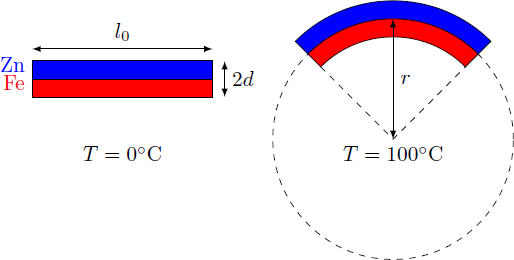# Bimetallic strip

A metal strip of length $l_0 = 20 \,\text{cm}$ consists of a layer of iron (Fe) and a layer of zinc (Zn), which are welded together with each having a thickness of $d = 2\,\text{mm}$. At a temperature of $T = 0\, ^\circ\text{C}$, the bimetallic strip is completely straight.

What is the radius of the curvature $r$ of the strip at a temperature of $T = 100\, ^\circ\text{C}?$

The respective thermal expansion coefficients of zinc and iron are

$\alpha_\text{Zn} = 3\cdot 10^{-5}/^\circ\text{C},\quad \alpha_\text{Fe} = 1\cdot 10^{-5}/^\circ\text{C}.$×